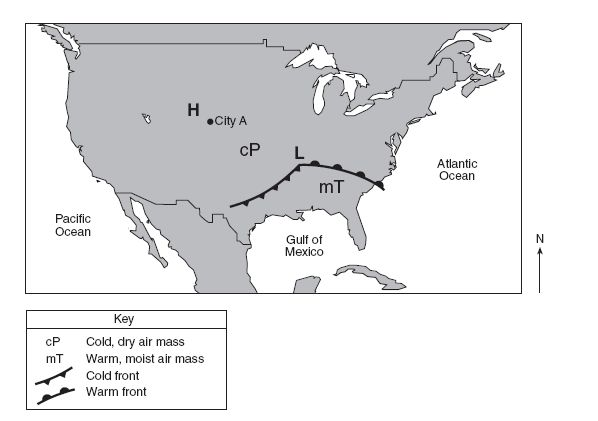# 604 Post Unit 2 Test

20 Questions | Total Attempts: 125SettingsThis test is to gauge your knowledge of topics surrounding weather.

Questions and Answers
• 1.
Earth’s weather is primarily caused by the
• A.

Drifting of Earth's crustal plates

• B.

Gravitational attraction of the Moon

• C.

Uneven heating of Earth's surface

• D.

Changing between Earth and the Sun

• 2.
Weather forecasts are more accurate today than in the past due to
• A.

Global warming

• B.

Air-quality control

• C.

Plate tectonics

• D.

Use of images from space

• 3.
Which process must occur for clouds to form?
• A.

Erosion

• B.

Conduction

• C.

Condensation

• D.

Precipitation

• 4.
The weather map below shows the locations of a high-pressure center (H) and a low-pressure center (L), air masses cP and mT, and city A. Explain why city A has clear sky conditions. 
• 5.
Oceans, glaciers, lakes, and rivers are part of Earth’s
• A.

Atmosphere

• B.

Hemisphere

• C.

Hydrosphere

• D.

Lithosphere

• 6.
Base your answers to the following questions on the information below and on your knowledge of science. The diagram shows a person observing a thunderstorm located several kilometers away. The person hears the thunder several seconds after seeing the lightning. Identify a form of energy, other than light and sound, that is often present during a thunderstorm. 
• 7.
A wet shirt is put on a clothesline to dry on a sunny day. The shirt dries because water molecules
• A.

Gain heat energy and condense

• B.

Gain heat energy and evaporate

• C.

Lose heat energy and condense

• D.

Lose heat energy and evaporate

• 8.
How should the students be placed in the room to represent the arrangement of particles in a solid? 
• 9.
What instruction should be given to the 20 student “particles” to show the addition of heat to the “solid sample of matter”? (Note: The solid is simply being heated; it should not melt.) 
• 10.
What instruction should be given to the 20 student “particles” to represent a liquid? 
• 11.
During which phase change is heat energy absorbed by a substance?
• A.

Liquid to gas

• B.

Gas to solid

• C.

liquid to solid

• D.

Gas to liquid

• 12.
The diagram below shows a Bunsen burner heating a beaker of water on a  beaker stand.  The arrows represent the transfer of heat energy in the water. Which process is primarily responsible for the transfer of heat indicated by the  arrows in the beaker of water?
• A.

Conduction

• B.

Convection

• C.

Radiation

• D.

Condensation

• 13.
Base your answers to the following question on the diagram below and on your knowledge of science. The diagram shows a model of the water cycle. Explain why the level of the muddy water will decrease slightly if the model is left in a sunny location for several days. 
• 14.
The graph below shows the heating curve for substance X. At approximately which temperature does a phase change begin?
• A.

- 30°C

• B.

-10°C

• C.

0°C

• D.

18°C

• 15.
Base your answers to questions 60 and 61 on the diagram below and on your knowledge of science. The diagram shows a sequence of events. The ball and ring are made of the same metal. Describe what can be done so that the metal ball will again go through the ring.
• 16.
The average daily air temperature at a certain location increases when there is a seasonal increase in the number of daylight hours. Which graph best shows this change?
• A.

• B.
• C.
• D.
• 17.
Base your answers to the question on the graph below and on your knowledge of science. The graph shows the average monthly air temperatures for three different locations, A, B, and C. Where is location C most likely located?
• A.

Southern Hemisphere

• B.

New York State

• C.

Equator

• D.

North Pole

• 18.
The diagram below shows milk being poured into a measuring cup. Which property of the milk can be directly measured using the cup?
• A.

Mass

• B.

Density

• C.

Solubility

• D.

Volume

• 19.
The diagram below shows a tall beaker with four different liquids and their densities. If a ball that has a density of 1.73 g/cm3 is placed in the beaker, where will the ball come to rest?
• A.

On top of liquid A

• B.

Between liquids B and C

• C.

Between liquids C and D

• D.

On the bottom of the beaker

• 20.
The tiny particles that make up all matter are called
• A.

Genes

• B.

Atoms

• C.

Minerals

• D.

Cells

Related Topics